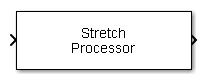# Stretch Processor

Stretch processor for linear FM waveforms

• Library:
• Phased Array System Toolbox / Detection

•## Description

The Stretch Processor block applies stretch processing on a linear FM waveform. Also known as dechirping, stretch processing is an alternative to matched filtering for linear FM waveforms.

## Ports

### Input

expand all

Input signal, specified as an M-by-P complex-valued array. M is the number of samples and P is the number of pulses.

The size of the first dimension of the input matrix can vary to simulate a changing signal length. A size change can occur, for example, in the case of a pulse waveform with variable pulse repetition frequency.

Data Types: `double`
Complex Number Support: Yes

Pulse repetition frequency of current pulse, specified as a positive scalar.

#### Dependencies

To enable this port, set the Specify PRF as parameter to `Input port`.

Data Types: `double`

### Output

expand all

Stretch processed signal, returned as an M-by-P complex-valued array. M is the number of samples and P is the number of pulses.

Data Types: `double`
Complex Number Support: Yes

## Parameters

expand all

Sample rate of the output waveform, specified as a positive scalar. The ratio of Sample rate (Hz) to each element in the Pulse repetition frequency (Hz) vector must be an integer. This restriction is equivalent to requiring that the pulse repetition interval is an integral multiple of the sample interval.

The duration of each pulse, specified as a positive scalar. Set the product of Pulse width (s) and Pulse repetition frequency to be less than or equal to one. This restriction ensures that the pulse width is smaller than the pulse repetition interval. Units are in seconds.

Example: `300e-6`

Source of PRF value for the stretch processor, specified as `Property`, `Auto`, or `Input port`. When set to `Property`, the Pulse repetition frequency (Hz) parameter sets the PRF. When set to ```Input port```, pass in the PRF using the `PRF` input port. When set to `Auto`, PRF is computed from the number of rows in the input signal.

.

Pulse repetition frequency, PRF, specified as a positive scalar. Units are in Hertz. Set this parameter to the same value set in any `Waveform` library block used in the simulation.

#### Dependencies

To enable this parameter, set the Specify PRF as parameter to `Property`.

Slope of the linear FM sweeping as a scalar, specified as a scalar. Units are in Hertz per second.

Example: `1e3`

FM sweep interval, specified as `Positive` or `Symmetric`. If you set this parameter value to `Positive`, the waveform sweeps the frequency bandwidth between 0 and B, where B is the frequency bandwidth. If you set this parameter value to `Symmetric`, the waveform sweeps in the interval between –B/2 and B/2.

Signal propagation speed, specified as a real-valued positive scalar. The default value of the speed of light is the value returned by `physconst('LightSpeed')`. Units are in meters per second.

Example: `3e8`

Data Types: `double`

Center of ranges of interest, specified as a positive scalar. The reference range must be within the unambiguous range of one pulse. Units are in meters.

Example: `10e3`

Span of ranges of interest, specified as a positive scalar. The span of ranges is centered on the range specified by the Reference range (m) parameter. Units are in meters.

Example: `1e3`

Source of simulation sample time, specified as ```Derive from waveform parameters``` or `Inherit from Simulink engine`. When set to `Derive from waveform parameters`, the block runs at a variable rate determined by the PRF of the selected waveform. The elapsed time is variable. When set to `Inherit from Simulink engine`, the block runs at a fixed rate so the elapsed time is a constant.

#### Dependencies

To enable this parameter, select the Enable PRF selection input parameter.

The format of the output signal, specified as `Pulses` or `Samples`.

If you set this parameter to `Samples`, the output of the block consists of multiple samples. The number of samples is the value of the Number of samples in output parameter.

If you set this parameter to `Pulses`, the output of the block consists of multiple pulses. The number of pulses is the value of the Number of pulses in output parameter.

Number of samples in the block output, specified as a positive integer.

Example: `1000`

#### Dependencies

To enable this parameter, set the Output signal format parameter to `Samples`.

Data Types: `double`

Number of pulses in the block output, specified as a positive integer.

Example: `2`

#### Dependencies

To enable this parameter, set the Output signal format parameter to `Pulses`.

Data Types: `double`

Select this parameter to enable the `PRF` output port.

#### Dependencies

To enable this parameter, set Output signal format to `Pulses`.

Block simulation, specified as `Interpreted Execution` or `Code Generation`. If you want your block to use the MATLAB® interpreter, choose `Interpreted Execution`. If you want your block to run as compiled code, choose ```Code Generation```. Compiled code requires time to compile but usually runs faster.

Interpreted execution is useful when you are developing and tuning a model. The block runs the underlying System object™ in MATLAB. You can change and execute your model quickly. When you are satisfied with your results, you can then run the block using ```Code Generation```. Long simulations run faster with generated code than in interpreted execution. You can run repeated executions without recompiling, but if you change any block parameters, then the block automatically recompiles before execution.

This table shows how the Simulate using parameter affects the overall simulation behavior.

When the Simulink® model is in `Accelerator` mode, the block mode specified using Simulate using overrides the simulation mode.

Acceleration Modes

 Block Simulation Simulation Behavior `Normal` `Accelerator` `Rapid Accelerator` `Interpreted Execution` The block executes using the MATLAB interpreter. The block executes using the MATLAB interpreter. Creates a standalone executable from the model. `Code Generation` The block is compiled. All blocks in the model are compiled.